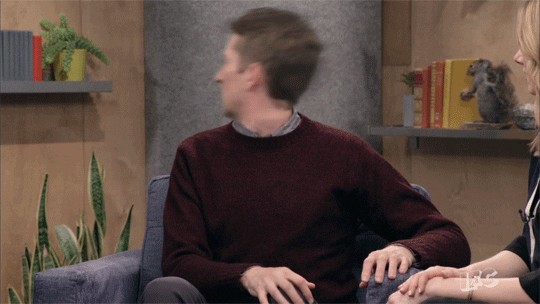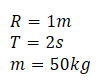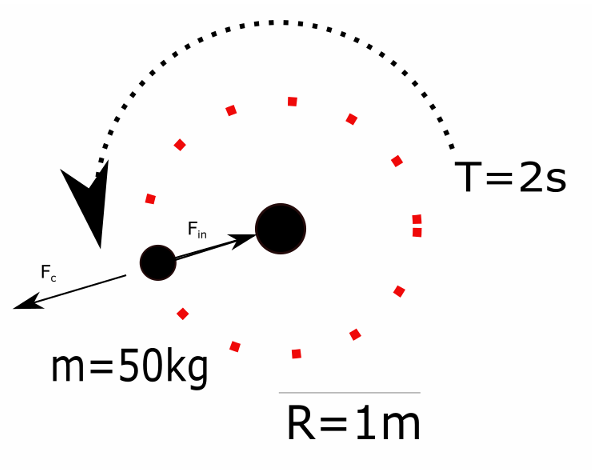top of page
Search

# Circular motion tutorialKey concepts:

Circular motion

Centrifugal force

Newton's laws

Did your head feel like ^^this^^ when your teacher explained centrifugal force? Circular motion is tricky, so let's walk through how it works (without making your head spin!)

Question 1:

"A playground carousel has a radius of 1 meter and makes one whole turn every 2 seconds. What is the centrifugal force experienced by a child with a mass of 50kg riding it?"

To answer this question, we need:

1. A sketch of the situation we are trying to describe.

2. The formula for centrifugal force.

1. A sketch of the situation we are trying to describe.TIP: Look, I know the sketch above is pretty bad. Yours will probably be better, but even if it isn't, don't feel too bad. Making a sketch should ALWAYS be the first thing you do when solving ANY problem in Math and Physics. It lets you see what the heck is even going on and can often tell you how to get started. Anyways, let's keep going:

We have definedThese symbols represent the radius of the carousel, how long it takes to make one revolution and the mass of the child respectively.

Now on to 2.

2. The formula for centrifugal force.Let us try to understand what the equation says in plain English:

"Imagine that you are in a situation, where you are rotating around some center. Then how much you feel like you are getting pushed away from the center depends on your mass, how fast you are going and how far away you are from the center."

Ok, that seems reasonable enough to anyone who has been in a carousel, a rollercoaster or a car that is turning sharply.

In our case, we don't actually have the speed of the child, at least not directly. Fortunately, we can calculate it. We know that a person on the carousel covers a distance every time they make one round trip. We also know that a round trip takes T=2s. Therefore we can say that:Let us use this to rewrite the formula for centrifugal force:Before we plug in the numbers, let us check that it makes sense. The equation now tells us that:

"The force you experience when rotating gets larger if your mass increases or if the radius of the carousel gets bigger. It also increases dramatically if you make the rotation time shorter."

Ok that seems reasonable as well. If you have ever been on a carousel that is going really fast, you know that it does not take long to make a round trip. Also the faster you go, the more you feel like you are being pulled off the carousel.

Let us plug in the numbers:Is 493N a lot of force? How big is this compared to other forces we are used to experiencing? Let us try to calculate the gravitational force on the child:Interesting! The outwards force due to rotation is almost the same as the downwards force due to gravity!

Question 2:

"As part of a game, a camp counselor is swinging a 50kg child around in a circle with a radius of 1m. They make one rotation every 2 seconds. With how much force must the camp counselor pull inwards to avoid losing their grip on the child?"

1. A sketch of the situation we are trying to model

2. A formula that tells us with how much force the child is being pulled out and away from the counselor.

1. A sketch of the situation we are trying to modelHmm if we look at this drawing, it is very similar to the situation in Question 1! We can therefore say thatThe centrifugal force is effectively pulling the child away from the counselor with a force of 493N. This means that the counsellor must pull inwards with exactly 493N to keep the child rotating 1m away and going around once every 2 seconds.

Here is the important point:

"If you have circular motion, there will always be a certain centrifugal force, Fc, pulling the rotating object away from the center. If the motion is steady then there must also be an equally large force pulling it in!"

Question 3

"Suppose we have a frictionless table. On top of it, we have a block with a mass of 1 kg. Via a string running through a hole in the middle of the table, it is attached to another block with a mass of 5kg, which is hanging straight down. If the first block is given a speed of 5m/s, it will start to move around in a circle. What will be the radius of this circle?"

Hmm, it is one of those wordy problems, huh? Don't worry, we will go through the usual steps and figure it out! We need:

1. A drawing of the situation we are trying to model.

2. An expression for the centrifugal force on the first block pulling it away from the middle.

3. An expression for the force pulling the first block in towards the middle.

1. A drawing of the situation we are trying to model.Perfect! Now we can actually see what is going on. The small block on top is going around in a circle, but is being kept in place by the force from the string. Its force comes from the weight of the big block.

Let us make some predictions:

a) We predict that making M bigger will make r smaller. After all, if there is more force pulling m in towards the middle, the orbit radius should be smaller!

b) If we increase v, then r should become bigger. It makes sense that the faster m moves, the more it gets pulled outwards.

Let us now take care of 2.

2. An expression for the centrifugal force on the first block pulling it away from the middle.

We can just use the centrifugal force formula: